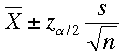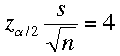SEARCH HOMEMath Central Quandaries & QueriesIf a population has standard deviation 20, what is the minimum sample size to be 95% confident that the error should be accurate to within 4?Hi,

If you can assume that the population is approximately normal then the formula for the confidence limits isSince you want a confidence interval with an error of ±4 you haveYou know that s = 20 and α = 0.5, hence you can solve for n.

HarleyMath Central is supported by the University of Regina and The Pacific Institute for the Mathematical Sciences.